# Mathematics Support

### The Real Number System

Magnitude and Scientific Notation

Rational and Irrational Numbers

## Magnitude and Scientific Notation

### Students will be able to...

• Use numbers expressed in the form of a single digit times an integer power of 10 to estimate very large or very small quantities, and to express how many times as much one is than the other.
• Know and apply the properties of integer exponents to generate equivalent numerical expressions.
• Perform operations with numbers expressed in scientific notation, including problems where both decimal and scientific notation are used. Use scientific notation and choose units of appropriate size for measurements of very large or very small quantities.

The Common Core State Standards require a balance of three fundamental components that result in rigorous mathematics acquisition: deep conceptual understanding, procedural skill, and mathematical applications and modeling.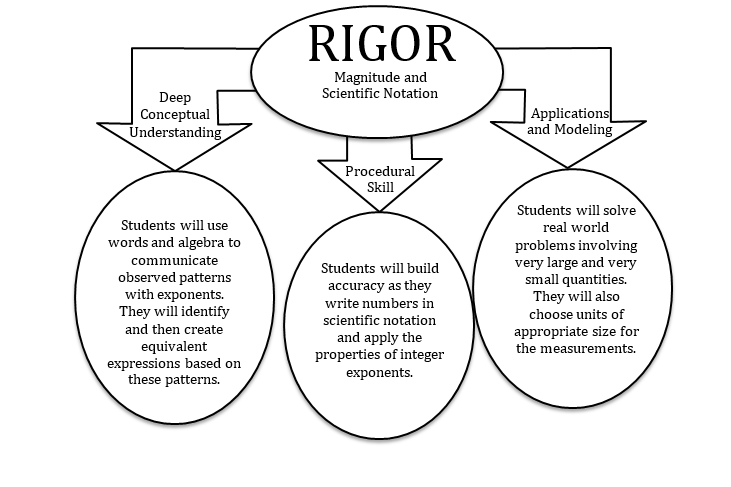### In school, your child will…

•  Use numbers expressed in the form of a single digit times an integer power of 10 to estimate very large or very small quantities, and to express how many times as much one is than the other.• Know and apply the properties of integer exponents to generate equivalent numerical expressions.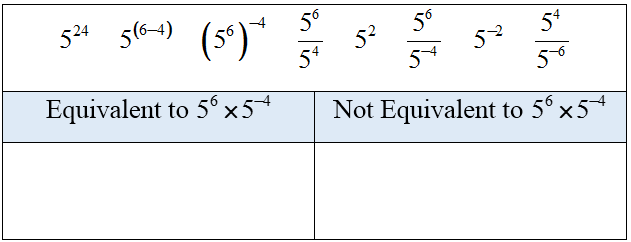• Perform operations with numbers expressed in scientific notation, including problems where both decimal and scientific notation are used. Use scientific notation and choose units of appropriate size for measurements of very large or very small quantities.

### At home, your child can…

• Explore more about how scientific notation is used in the real world by visiting Mass of the Planets.
• Examine the Richter scale and see how scientific notation is used in describing the magnitude of earthquakes. Visit the CK12 PLIX (Play Learn Interact Xplore): Scientific Notation: Richter Scale Exponents. To access the PLIX, you will need to create a free user account.
• Investigate the populations of countries that are being discussed in current events. Record the populations in scientific notation and express how many times as much one is than the other.

*Additional Practice links support C2.0 content, but may use vocabulary or strategies not emphasized by MCPS.

## Rational and Irrational Numbers

### Students will be able to...

• Know that numbers that are not rational are called irrational. Understand informally that every number has a decimal expansion; for rational numbers show that the decimal expansion repeats eventually, and convert a decimal expansion which repeats eventually into a rational number.
• Use square root and cube root symbols to represent solutions to equations of the form x2 = p and x3 = p, where p is a positive rational number. Evaluate square roots of small perfect squares and cube roots of small perfect cubes. Know that √2 is irrational.
• Use rational approximations of irrational numbers to compare the size of irrational numbers, locate them approximately on a number line diagram, and estimate the value of expressions.

The Common Core State Standards require a balance of three fundamental components that result in rigorous mathematics acquisition: deep conceptual understanding, procedural skill, and mathematical applications and modeling.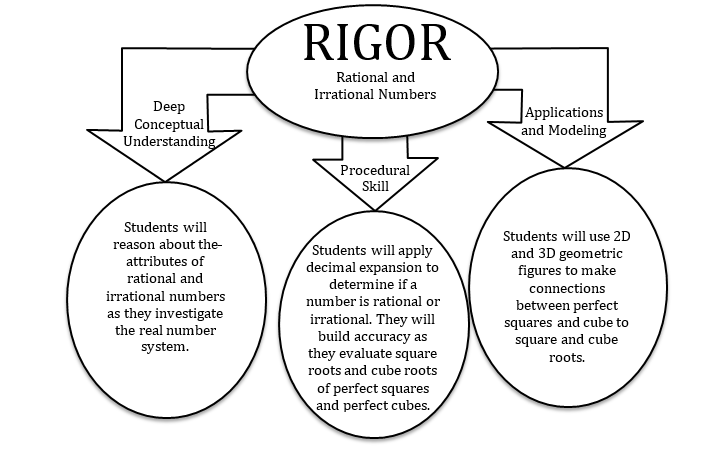### In school, your child will…

• Know that numbers that are not rational are called irrational. Understand informally that every number has a decimal expansion; for rational numbers show that the decimal expansion repeats eventually, and convert a decimal expansion which repeats eventually into a rational number.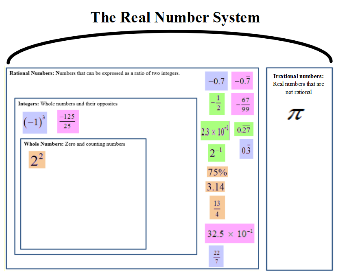• Use square root and cube root symbols to represent solutions to equations of the form x2 = p and x3 = p, where p is a positive rational number. Evaluate square roots of small perfect squares and cube roots of small perfect cubes. Know that √2 is irrational.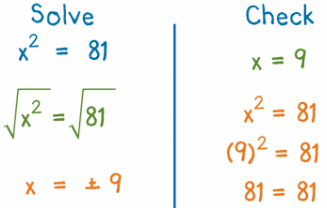• Use rational approximations of irrational numbers to compare the size of irrational numbers, locate them approximately on a number line diagram, and estimate the value of expressions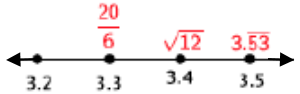### At home, your child can...

• Use a standard clock, to change the numbers on the face to the perfect squares. To reinforce square roots of perfect squares.
• Use graph paper, to approximate and draw perfect squares to determine if a number is rational or irrational.  Have your child find the area, using length times width and find the perfect square or approximate non-perfect squares.